• 主要为大家详细介绍了python实现基于SVM手写数字识别功能，具有一定的参考价值，感兴趣的小伙伴们可以参考一下
• python实现手写数字识别

千次阅读 2021-01-02 12:50:11

手写数字识别是一个经典的机器学习问题，通过识别手写体图片来判断数字

因为数字类别是0——9，所以是十分类问题

本文以KNN算法为例，来实现手写数字的识别

低维的手写数字识别

digits是一个Bunch类型的类字典对象，我们可以利用索引来调用它

调用

from sklearn import datasets

索引

digits有5个部分：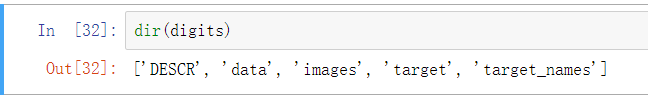data：数据，其中每个元素是64维的向量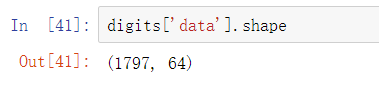images：图像，其中每个元素是8×8的矩阵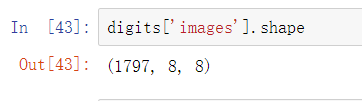target：每个数据对应的标签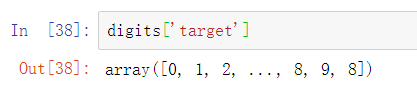target_names：所有的类别标签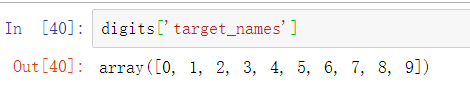以第0个元素为例：

64维的向量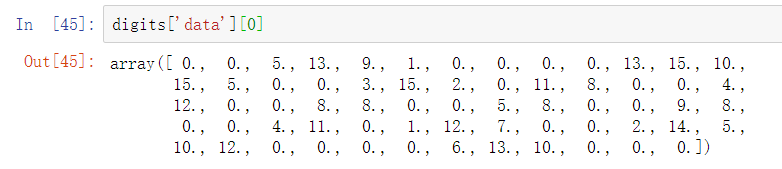8×8的矩阵，可以大致看出数字 ‘0’ 的轮廓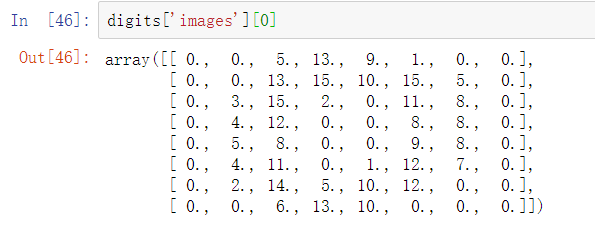用plt.imshow()可以将images可视化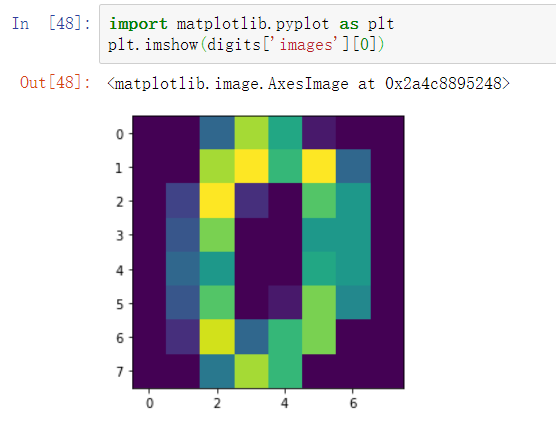数据的类别标签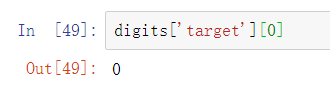模型的预测结果：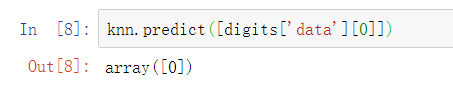使用KNN来进行训练和预测

对数据集进行划分，使用训练集训练knn，再使用测试集测试性能

from sklearn.model_selection import train_test_split
from sklearn.neighbors import KNeighborsClassifier

X_train, X_test, y_train, y_test = train_test_split(digits['data'], digits['target'])
knn = KNeighborsClassifier()
knn.fit(X_train, y_train)
knn.score(X_test, y_test)

score函数返回的是模型在测试集上的正确率，使用形式为：

model.score(X_test, y_test)

可以看到正确率是很高的：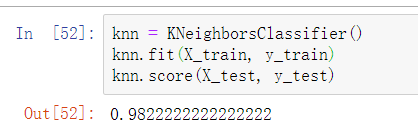高维的手写数字识别

导入数据集

从本地导入一个mnist.npy文件

import numpy as np
x_train, x_test, y_train, y_test = np.load('data/mnist/mnist.npy', allow_pickle = True)

训练集的shape：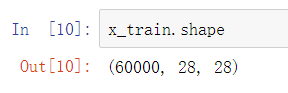测试集的shape：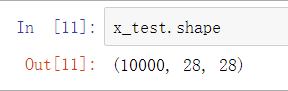reshape数据集

查看数据的shape，可以看到每个数据都是一个28×28的矩阵

因为无法直接对矩阵进行运算，所以需要把矩阵转换为784的向量

# reshape训练集
n_samples, n1, n2 = x_train.shape
x_train = x_train.reshape(n_samples, n1*n2).astype(np.float32)
# reshape测试集
n_samples_test, n1_test, n2_test = x_test.shape
x_test = x_test.reshape(n_samples_test, n1_test*n2_test).astype(np.float32)

此时每个数据都变成了784的向量：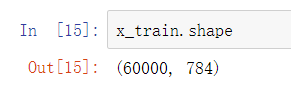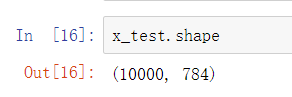特征降维

因为训练集有60000个数据，需要较长的时间，可以将维度降低来减少运行时间

使用PCA主成分分析，降维到64维

# 特征降维
from sklearn.decomposition import PCA
pca = PCA(n_components = 64)
decom_x_train = pca.fit_transform(x_train)
decom_x_test = pca.transform(x_test)

因为之前把第5行的pca.transform写成了pca.fit_transform，导致正确率特别低，修改之后就好了

再使用降维后的训练集样本进行训练

knn = KNeighborsClassifier()
knn.fit(decom_x_train, y_train)
knn.score(decom_x_test, y_test)

在测试集上的正确率：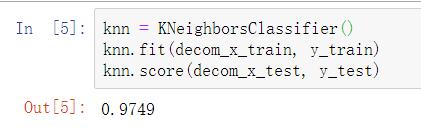展开全文机器学习 分类算法
• 包含mnist手写数字数据集、可视化展示功能、适用于Python3、重要代码注释
• 主要为大家详细介绍了python神经网络编程实现手写数字识别，文中示例代码介绍的非常详细，具有一定的参考价值，感兴趣的小伙伴们可以参考一下
• 一份基于cnn的手写数字识别的代码，供大家参考，具体内容如下 # -*- coding: utf-8 -*- import tensorflow as tf from tensorflow.examples.tutorials.mnist import input_data # 加载数据集 mnist = input_data....
• （1）认识MNIST数据集的数据格式，对...（2）利用python语言从零开始搭建多层感知机网络； （3） 通过调整参数提高多层感知机网络的准确度，并对实验结果进行评估； （4）程序的语句要求有注释，以增强程序可读性。
• python实现手写数字识别（小白入门）

万次阅读 多人点赞 2020-05-16 18:34:47
手写数字识别（小白入门） 今早刚刚上了节实验课，关于逻辑回归，所有手有点刺挠就想发个博客，作为刚刚入门的小白，看到代码运行成功就有点小激动，这个实验没啥含金量，所以路过的大牛不要停留，我怕你们吐槽。 ...

手写数字识别（小白入门）

今早刚刚上了节实验课，关于逻辑回归，所以手有点刺挠就想发个博客，作为刚刚入门的小白，看到代码运行成功就有点小激动，这个实验没啥含金量，所以路过的大牛不要停留，我怕你们吐槽哈哈。

实验结果：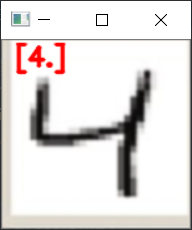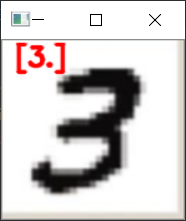1.数据预处理

其实呢，原理很简单，就是使用多变量逻辑回归，将训练28*28图片的灰度值转换成一维矩阵，这就变成了求784个特征向量1个标签的逻辑回归问题。代码如下：

#数据预处理
MTrain, NTrain = np.shape(trainData)  #行列数
print("训练集：",MTrain,NTrain)
xTrain = trainData[:,1:NTrain]
xTrain_col_avg = np.mean(xTrain, axis=0) #对各列求均值
xTrain =(xTrain- xTrain_col_avg)/255  #归一化
yTrain = trainData[:,0]

2.训练模型

对于数学差的一批的我来说，学习算法真的是太太太扎心了，好在具体算法封装在了sklearn库中。简单两行代码即可完成。具体参数的含义随随便便一搜到处都是，我就不班门弄斧了，每次看见算法除了头晕啥感觉没有。

model = LogisticRegression(solver='lbfgs', multi_class='multinomial', max_iter=500)
model.fit(xTrain, yTrain)

3.测试模型，保存

接下来测试一下模型，准确率能达到百分之90，也不算太高，训练数据集本来也不是很多。
为了方便，所以把模型保存下来，不至于运行一次就得训练一次。

#测试模型
MTest,NTest = np.shape(testData)
print("测试集：",MTest,NTest)
xTest = testData[:,1:NTest]
xTest = (xTest-xTrain_col_avg) /255   # 使用训练数据的列均值进行处理
yTest = testData[:,0]
yPredict = model.predict(xTest)
errors = np.count_nonzero(yTest - yPredict) #返回非零项个数
print("预测完毕。错误：", errors, "条")
print("测试数据正确率:", (MTest - errors) / MTest)

'''================================='''
#保存模型

# 创建文件目录
dirs = 'testModel'
if not os.path.exists(dirs):
os.makedirs(dirs)
joblib.dump(model, dirs+'/model.pkl')
print("模型已保存")

4.调用模型

既然模型训练好了，就来放几张图片调用模型试一下看看怎么样
导入要测试的图片，然后更改大小为28*28，将图片二值化减小误差。
为了让结果看起来有逼格，所以最后把图片和识别数字同实显示出来。

import  cv2
import numpy as np
from sklearn.externals import joblib

map=cv2.imread(r"C:\Users\lenovo\Desktop\[DX6@[C$%@2RS0R2KPE[W@V.png") GrayImage = cv2.cvtColor(map, cv2.COLOR_BGR2GRAY) ret,thresh2=cv2.threshold(GrayImage,127,255,cv2.THRESH_BINARY_INV) Image=cv2.resize(thresh2,(28,28)) img_array = np.asarray(Image) z=img_array.reshape(1,-1) '''================================================''' model = joblib.load('testModel'+'/model.pkl') yPredict = model.predict(z) print(yPredict) y=str(yPredict) cv2.putText(map,y, (10,20), cv2.FONT_HERSHEY_SIMPLEX,0.7,(0,0,255), 2, cv2.LINE_AA) cv2.imshow("map",map) cv2.waitKey(0) 5.完整代码 test1.py import numpy as np from sklearn.linear_model import LogisticRegression import os from sklearn.externals import joblib #数据预处理 trainData = np.loadtxt(open('digits_training.csv', 'r'), delimiter=",",skiprows=1)#装载数据 MTrain, NTrain = np.shape(trainData) #行列数 print("训练集：",MTrain,NTrain) xTrain = trainData[:,1:NTrain] xTrain_col_avg = np.mean(xTrain, axis=0) #对各列求均值 xTrain =(xTrain- xTrain_col_avg)/255 #归一化 yTrain = trainData[:,0] '''=================================''' #训练模型 model = LogisticRegression(solver='lbfgs', multi_class='multinomial', max_iter=500) model.fit(xTrain, yTrain) print("训练完毕") '''=================================''' #测试模型 testData = np.loadtxt(open('digits_testing.csv', 'r'), delimiter=",",skiprows=1) MTest,NTest = np.shape(testData) print("测试集：",MTest,NTest) xTest = testData[:,1:NTest] xTest = (xTest-xTrain_col_avg) /255 # 使用训练数据的列均值进行处理 yTest = testData[:,0] yPredict = model.predict(xTest) errors = np.count_nonzero(yTest - yPredict) #返回非零项个数 print("预测完毕。错误：", errors, "条") print("测试数据正确率:", (MTest - errors) / MTest) '''=================================''' #保存模型 # 创建文件目录 dirs = 'testModel' if not os.path.exists(dirs): os.makedirs(dirs) joblib.dump(model, dirs+'/model.pkl') print("模型已保存") 运行结果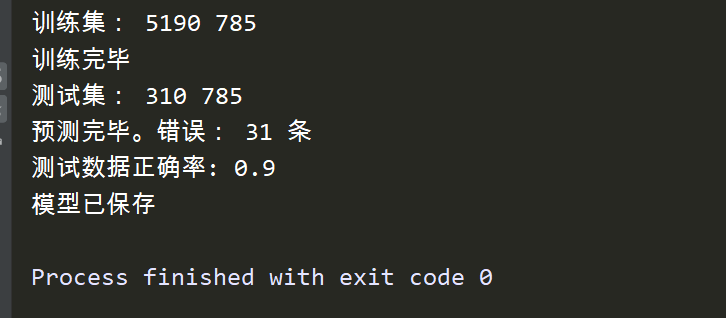test2.py import cv2 import numpy as np from sklearn.externals import joblib map=cv2.imread(r"C:\Users\lenovo\Desktop\[DX6@[C$%@2RS0R2KPE[W@V.png")
GrayImage = cv2.cvtColor(map, cv2.COLOR_BGR2GRAY)
ret,thresh2=cv2.threshold(GrayImage,127,255,cv2.THRESH_BINARY_INV)
Image=cv2.resize(thresh2,(28,28))
img_array = np.asarray(Image)
z=img_array.reshape(1,-1)

'''================================================'''

yPredict = model.predict(z)
print(yPredict)
y=str(yPredict)
cv2.putText(map,y, (10,20), cv2.FONT_HERSHEY_SIMPLEX,0.7,(0,0,255), 2, cv2.LINE_AA)
cv2.imshow("map",map)
cv2.waitKey(0)

提供几张样本用来测试：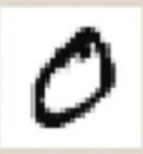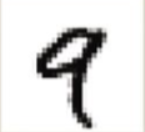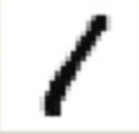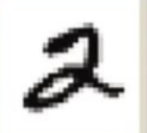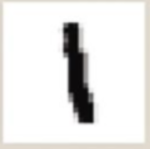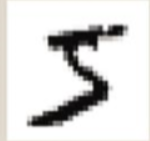实验中还有很多地方需要优化，比如数据集太少，泛化能力太差，用样本的数据测试正确率挺高，但是用我自己手写的字正确率就太低了，可能我字写的太丑，哎，还是自己太菜了，以后得多学学算法了。

训练好的模型放在了上面。最后我也把数据集放到这儿。链接：https://pan.baidu.com/s/1PfQ5Jp3A8eN4SxFnA12-1Q
提取码：tpy6

展开全文机器学习 python 人工智能 计算机视觉
• 基于BP神经网络和sklearn的digit数据集编写的手写数字识别demo。带有GUI手写画板，同时还可以根据需要保存手写数字的数据。手写数字识别
• 本资源为纯python实现mnist手写识别的代码，为作者本人所写，供深度学习初学者共同交流探讨，欢迎二次创作，网络为三层，可达到97%上准确率，模型可以选择多种训练方式，学习率，激活函数，损失函数等我都写了相关...
• 本文使用python基于TensorFlow设计手写数字识别算法，并编程实现GUI界面，构建手写数字识别系统。这是本人的本科毕业论文课题，当然，这个也是机器学习的基本问题。本博文不会以论文的形式展现，而是以编程实战完成...
• 今年来人工智能火的不行，大家都争相学习机器学习，作为学习大军中的一员，我觉得最好的学习方法就是用python把机器学习算法实现一遍，下面我介绍一下用逻辑回归实现手写字体的识别。 逻辑回归知识点回顾 ​ ...

​ 有一句话说得好，要有造轮子的技术和用轮子的觉悟，今年来人工智能火的不行，大家都争相学习机器学习，作为学习大军中的一员，我觉得最好的学习方法就是用python把机器学习算法实现一遍，下面我介绍一下用逻辑回归实现手写字体的识别。

逻辑回归知识点回顾

​ 线性回归简单又易用 hθ(x)=θTx h θ ( x ) = θ T x $h_\theta(x)=\theta^Tx$，可以进行值的预测，但是不擅长分类。在此基础上进行延伸，把预测的结果和概率结合起来就可以做分类器了，比如预测值大于0.5，则归为1类，否则就归为0类，这个就是逻辑回归算法了。逻辑回归主要解决的就是二分类问题，比如判断图片上是否一只猫，邮件是否垃圾邮件等。

​ 由于逻辑回归的结果是概率值，为0-1之间，因此需要在线性回归的结果上增加一次运算，使得最后的预测结果在0到1之间。

Sigmoid函数，表达式为： g(z)=11+ez g ( z ) = 1 1 + e − z $g(z)=\frac{1}{1+e^{-z}}$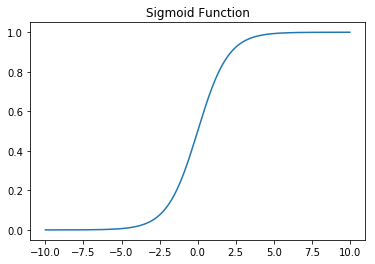​ sigmoid的输出在0和1之间，我们在二分类任务中，采用sigmoid的输出的是事件概率，也就是当输出满足满足某一概率条件我们将其划分正类。

结合了sigmoid函数，把线性回归的结果概率化，得到预测函数为 hθ(x(i))=g(θTx(i)) h θ ( x ( i ) ) = g ( θ T x ( i ) ) $h_\theta(x^{(i)})=g(\theta^Tx^{(i)})$ g(z)=11+ez g ( z ) = 1 1 + e − z $g(z)=\frac{1}{1+e^{-z}}$。当概率大于0.5时为1类，否则判定位0类。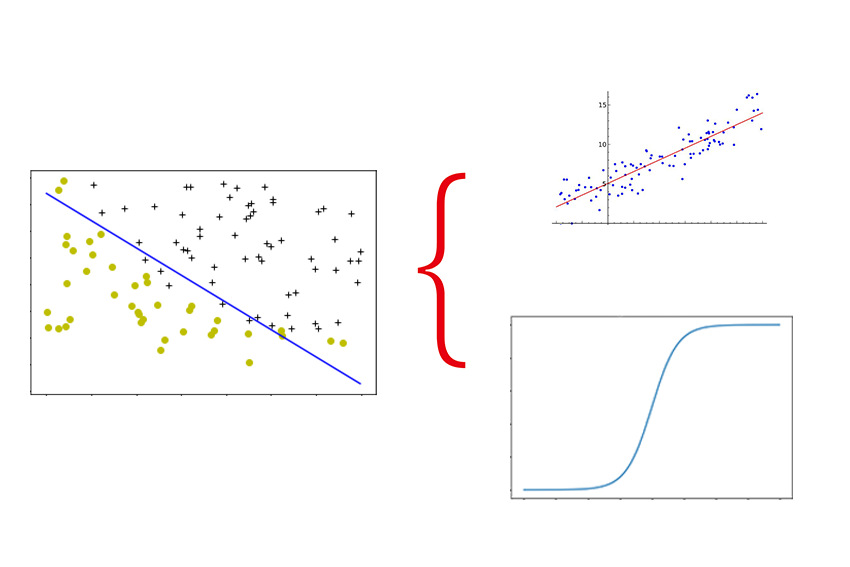接下来我们开始使用逻辑回归进行手写字体的识别！

查看手写字体数据

​ 此次使用的是5000条手写数字的数据。一条训练数据是20px*20px图片的数据，每一个像素点是代表灰度值。我们查看一下前100条手写数据，如下图：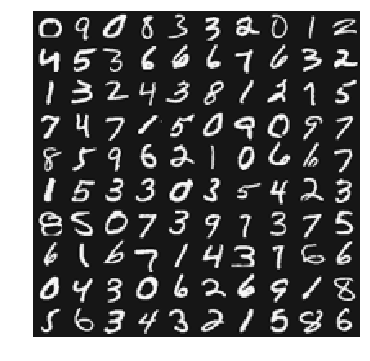数据集下载地址：

定义向量化的预测函数 hθ(x) h θ ( x ) $h_\theta(x)$

首先我们定义预测函数 hθ(x(i))=g(θ0+θ1x(i)1+θ2x(i)2+...+θkx(i)k) h θ ( x ( i ) ) = g ( θ 0 + θ 1 x 1 ( i ) + θ 2 x 2 ( i ) + . . . + θ k x k ( i ) ) $h_\theta(x^{(i)})=g(\theta_0+\theta_1x_1^{(i)}+\theta_2x_2^{(i)}+...+\theta_kx_k^{(i)})$ ,k是参数的个数。

写成向量化的形式为：

hθ(x(i))=g(θTx(i)) h θ ( x ( i ) ) = g ( θ T x ( i ) ) $h_\theta(x^{(i)})=g(\theta^Tx^{(i)})$ , g(z)=11+ez g ( z ) = 1 1 + e − z $g(z)=\frac{1}{1+e^{-z}}$ ，g(z)是激活函数（sigmoid function）

def h(mytheta,myX):
#expit是scipy库中向量化sigmoid函数的计算函数
return expit(np.dot(myX, mytheta))

预测函数中 θ θ $\theta$的值是未知的，接下来的任务就是要根据训练集，求解出 θ θ $\theta$的值。

计算代价函数(Cost Function)

在线性回归中的最小二乘法，求解参数 θ θ $\theta$就是最小化残差平方和，也就是代价函数 J(θ) J ( θ ) $J(\theta)$。代价函数 J(θ) J ( θ ) $J(\theta)$是度量预测错误的程度，逻辑回归的代价函数为对数似然函数，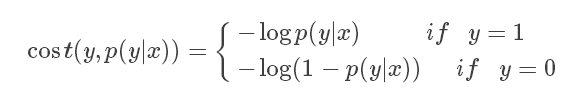等价于：

J(θ)=1mmi=1[y(i)log(hθ(x(i)))(1y(i))log(1hθ(x(i)))] J ( θ ) = 1 m ∑ i = 1 m [ − y ( i ) l o g ( h θ ( x ( i ) ) ) − ( 1 − y ( i ) ) l o g ( 1 − h θ ( x ( i ) ) ) ] $J(\theta)=\frac{1}{m}\sum_{i=1}^{m}[-y^{(i)}log(h_\theta(x^{(i)})) - (1 - y^{(i)})log(1 - h_{\theta}(x^{(i)}))]$

def computeCost(mytheta,myX,myy):
m = len(X) #5000
term1 = np.dot(-np.array(myy).T,np.log(h(mytheta,myX)))#shape(1,401)
term2 = np.dot((1-np.array(myy)).T,np.log(1-h(mytheta,myX)))#shape(1,401)
return float((1./m) * np.sum(term1 - term2) )

使得预测错误的程度最低，即使得 J(θ) J ( θ ) $J(\theta)$最小，此时我们把问题转为最优化问题。

下面介绍一种求解最优化的算法：梯度下降。从初始位置每次向梯度方向(就是下降速度最快的方向)迈一小步，一直走到最低点。想象你在下山，你朝着当前位置下降最快的方向走一步。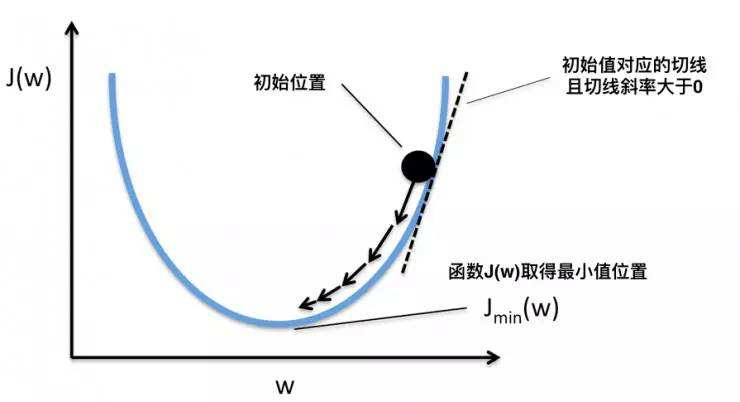计算梯度

经过数学运算可以得出 Jθ=1mmi=1(hθ(x(i))y(i)x(i)j) ∂ J ∂ θ = 1 m ∑ i = 1 m ( h θ ( x ( i ) ) − y ( i ) x j ( i ) ) $\frac{\partial J}{\partial \theta} =\frac{1}{m}\sum_{i=1}^m(h_\theta(x^{(i)})-y^{(i)}x_j^{(i)})$

m = myX.shape
beta = h(mytheta,myX)-myy.T #shape: (5000,1)
grad = (1./m)*np.dot(myX.T,beta) #shape: (401, 5000)

最小化 J(θ) J ( θ ) $J(\theta)$求解 θ θ $\theta$

这里我们使用的是高级算法，scipy中的optimize，求解最小化 J(θ) J ( θ ) $J(\theta)$ θ θ $\theta$的值。

from scipy import optimize

def optimizeTheta(mytheta,myX,myy):
result = optimize.fmin_cg(computeCost, fprime=costGradient, x0=mytheta, \
args=(myX, myy), maxiter=50, disp=False,\
full_output=True)
return result, result

One-VS-All多分类

逻辑回归擅长二分类问题，因此需要把多分类问题转换为多个二分类问题进行解决。如下图，首先把蓝色和绿色标记看为一类，红色记为一类，这样子就可以用预测函数 hθ(x) h θ ( x ) $h_\theta(x)$计算出新数据为红色类别的概率，同理也可以计算出新数据为蓝色、绿色类别的概率，选择概率最高的类别作为新数据的预测分类，这就是One-VS-All多分类算法。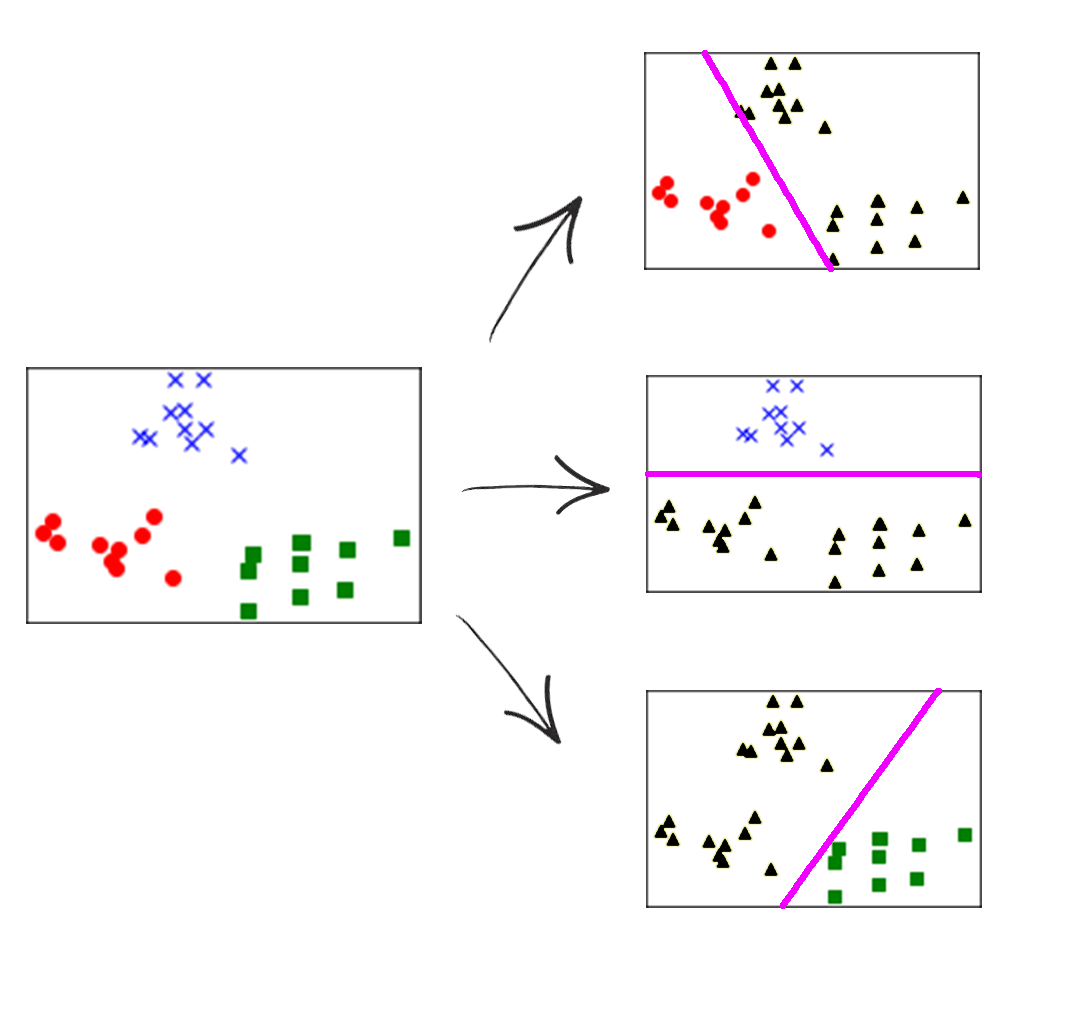def predictOneVsAll(myTheta,myrow):
classes =  + list(range(1,10))
hypots  = *len(classes)
for i in range(len(classes)):
hypots[i] = h(myTheta[:,i],myrow)
return classes[np.argmax(np.array(hypots))]

预测并计算准确率

计算测试集得到逻辑回归预测的准确度为 89.1%。抽看第1601个数据观察预测情况

predictOneVsAll(Theta,X)
#3
scipy.misc.toimage(X[1:].reshape(20,20).T)

画出第1601个数据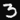逻辑回归的预测准确率是89.1%，那么以逻辑回归作为神经元的神经网络算法，是否会更加强大呢，我们下期见！

展开全文机器学习 Python 数据分析 数据挖掘
• 手写数字识别原始数据，0~9数字，32*32，贝叶斯代码实现手写体识别和大致出错率计算，可用于python学习实践。 github免费下载：...
• 用多元逻辑回归手写数字识别训练样本简介首先，可以用逻辑回归实现手写数字识别的多元分类，在ex3data.mat文件中保存着一个包含5000个培训考试训练数据集的手写数字，.mat格式表示这些训练数据集以矩阵格式保存，而...

前言

在之前的学习中，已经对神经网络的算法具体进行了学习和了解。现在，我们可以用python通过两种方法来实现手写数字的识别。这两种方法分别是多元逻辑回归和神经网络方法。

用多元逻辑回归手写数字识别

训练样本简介

首先，可以用逻辑回归实现手写数字识别的多元分类，在ex3data.mat文件中保存着一个包含5000个培训考试训练数据集的手写数字，.mat格式表示这些训练数据集以矩阵格式保存，而不是文本格式。训练集中有5000个训练样本，每个训练样本是一个20X20像素的灰度图像，每一个像素用一个float型数据表示当前位置的灰度强度。20X20像素形成一个400维向量，每一个训练样本形成一个单行矩阵，5000个训练样本构成一个5000X400的矩阵，每一个行向量代表手写数字图像的训练样本。矩阵可以如下表示：数据集第二部分包含一个5000维的矩阵, 代表输出值的标签，即0,1,2,3,4,5,6,7,8,9.

如下代码所示，首先导入算法实现所需要的python库

import matplotlib.pyplot as plt

import numpy as np

import scipy.io as scio

初始化一些参数

input_layer_size = 400 # 20x20 像素矩阵

num_labels = 10 # 手写数字 0,1,2,3,4,5,6,7,8,9

数据可视化

随机选取矩阵中的100行向量，并将这些函数传递到displayData函数中，通过调用此函数，我们可以显示出这些向量的灰度图像，具体实现如下所示：

载入数据

X = data['X']

y = data['y'].flatten()

m = y.size

绘图函数实现

绘图函数的实现如下所示，具体实现思路就是确定显示图像的像素大小，然后读取训练集数据，依次将其填充到设定好大小的像素矩阵中，具体可参考代码注释。

def display_data(x):

(m, n) = x.shape

# m = 100

# n = 400

# 设置每个数字的宽度与高度(像素)

example_width = np.round(np.sqrt(n)).astype(int)# example_width=20

example_height = (n / example_width).astype(int) #example_height=20

# 计算显示的行数与列数

display_rows = np.floor(np.sqrt(m)).astype(int) #display_rows=10

display_cols = np.ceil(m / display_rows).astype(int)#display_rows=10

# 单个图像之间的间隔

# 设置并初始化显示像素矩阵的大小211*211 ,1+(10*20+1)

# 将每个训练样本显示在矩阵中

curr_ex = 0

for j in range(display_rows):

for i in range(display_cols):

if curr_ex > m:

break

# 每次每行和每列读取一个20*20像素的数字，矩阵大小加21

# 实际上矩阵形式可以认为 10*10*400(20*20像素)

max_val = np.max(np.abs(x[curr_ex]))

pad + i * (example_width + pad) + np.arange(example_width)[:, np.newaxis]] = \

x[curr_ex].reshape((example_height, example_width)) / max_val

curr_ex += 1

if curr_ex > m:

break

# Display image

plt.figure()

plt.imshow(display_array, cmap='gray', extent=[-1, 1, -1, 1])

plt.axis('off')

最后，绘图实现效果如下矢量化逻辑回归

参考逻辑回归与正则化的相关知识，矢量化逻辑回归的算法实现代码如下所示：

def lr_cost_function(theta, X, y, lmd):

m = y.size

cost = 0

reg_theta = theta[1:]

hy = sigmoid(np.dot(X,theta))

cost = np.sum(-y * np.log(hy) - np.subtract(1, y) * np.log(np.subtract(1, hy))) / m + (lmd / (2 * m)) * np.sum(reg_theta * reg_theta)

grad = np.dot(X.T, np.subtract(hy, y)) / m

def sigmoid(z):

return 1/(1+np.exp(-z))

人为构造一些参数值，计算结果如下所示：

theta_t = np.array([-2, -1, 1, 2])

X_t = np.c_[np.ones(5), np.arange(1, 16).reshape((3, 5)).T/10]

y_t = np.array([1, 0, 1, 0, 1])

lmda_t = 3

cost, grad = lr_cost_function(theta_t, X_t, y_t, lmda_t)参数训练

在之前的代码中，已经实现了逻辑回归的梯度下降算法，利用训练样本，现在可以通过梯度下降算法训练参数了，其中，与二元分类不同的是，在本次代码中需要实现的是0-9的数字分类，与用0,1实现二元分类相比，更加复杂，所以在多元分类中，我们用多元逻辑回归算法实现多元分类时，通过0和1来区分每个数字是否识别成功，简而言之，相当于进行了10次二元逻辑回归，只不过每次的值会发生变化而已。具体实现代码如下所示：

def one_vs_all(X, y, num_labels, lmd):

(m, n) = X.shape

all_theta = np.zeros((num_labels, n + 1))

X = np.c_[np.ones(m), X]

for i in range(num_labels):

initial_theta = np.zeros((n + 1, 1))

iclass = i if i else 10

y_i = np.array([1 if x == iclass else 0 for x in y])

print('Optimizing for handwritten number {}...'.format(i))

def cost_func(t):

return lr_cost_function(t, X, y_i, lmd)

return lr_cost_function(t, X, y_i, lmd)

theta, *unused = opt.fmin_cg(cost_func, fprime=grad_func, x0=initial_theta, maxiter=100, disp=False,

full_output=True)

print('Done')

all_theta[i] = theta

return all_theta

调用one_vs_all()函数，可以看到通过梯度下降算法得到的参数矩阵如下所示，用多元逻辑回归算法实现一对多分类预测

现在，我们已经实现了多元逻辑回归算法，现在可以利用梯度下降算法获得的参数实现一对多分类的具体应用了，具体实现代码可以如下表示：

def predict_one_vs_all(all_theta, X):

m = X.shape

num_labels = all_theta.shape

p = np.zeros(m)

X = np.c_[np.ones(m), X]

result = np.dot(all_theta, X.T)

result = np.roll(result, -1, axis=0)

result = np.vstack([np.zeros(m), result])

p = np.argmax(result, axis=0)

return p

pred = predict_one_vs_all(all_theta, X)

print('Training set accuracy: {}'.format(np.mean(pred == y)*100))

经过计算，识别精确率如下所示：用神经网路实现手写数字识别

初始化一些参数

根据算法要求，首先需要初始化一些参数，具体代码如下所示：

theta1 = data['Theta1']

theta2 = data['Theta2']

计算神经网络算法识别效果的精确度

利用神经网络实现多元分类时，不再计算损失函数和梯度，利用输入层，隐藏层和输出层，我们可以直接利用神经网络实现手写数字识别的多元分类。具体实现代码如下所示：

def predict(theta1, theta2, x):

num_labels = theta2.shape

p = np.zeros(m)

x = np.c_[np.ones(m), x]

h1 = sigmoid(np.dot(x, theta1.T))

h1 = np.c_[np.ones(h1.shape), h1]

h2 = sigmoid(np.dot(h1, theta2.T))

p = np.argmax(h2, axis=1) + 1

return p

分析以上代码，我们可以发现这是有一个输入层(矩阵)，一个

隐藏层(由矩阵计算得到的)，由隐藏层计算得到的输出层(),最后，由输出层得到分类结果。

通过神经网络得到识别的精确率如下所示：展开全文用python写数字
• 博客：https://blog.csdn.net/weixin_45775701/article/details/109446515
• 利用贝叶斯分类器实现手写数字识别，要数据的可以发私信，有测试集和训练集。python
• 文章目录安装mahotas库智能提取阈值一、加载必要的库和一些基本函数二、定义hog算子来描述图片的特征三、根据已有数字类别标注的数据集来训练模型四、利用训练好的模型进行数字识别数据集下载和完整代码附录 ...opencv 数字识别
• 主要为大家详细介绍了机器学习python实战之手写数字识别，具有一定的参考价值，感兴趣的小伙伴们可以参考一下
• 写在前面其实我之前写过一个简单的识别手写数字的程序，但是因为逻辑比较简单，而且要求比较严苛，是在50x50大小像素的白底图上手写黑色数字，并且给的训练材料也不够多，导致准确率只能五五开。所以这一次准备写一...
• 主要为大家详细介绍了python实现识别手写数字python图像识别算法，具有一定的参考价值，感兴趣的小伙伴们可以参考一下
• 使用Python基于TensorFlow 卷积神经网络设计手写数字识别算法，并编程实现GUI 界面，构建手写数字识别系统。本系统界面设计友好，功能完善。通过测试，本识别系统对于较规范的手写体数字的识别达到了很好的识别效果...
• 主要介绍了详解python实现识别手写MNIST数字集的程序，小编觉得挺不错的，现在分享给大家，也给大家做个参考。一起跟随小编过来看看吧
• 该程序使用Python实现BP神经网络mnist手写数字识别。压缩包中给出训练好的权重以及偏移量的mat文件，在程序运行后输入mnist文件夹中图片路径的后缀就可以进行预测，如：4/mnist_test_4.png。压缩包给出训练集的mat...
• 机器学习入门KNN算法实现手写数字识别 基本上照搬了http://lib.csdn.net/article/opencv/30167的代码，只是改了一点bug和增加了一点功能 输入就是直接在一个512*512大小的白色画布上画黑线，然后转化为01矩阵，用...
• python softmax实现手写数字识别， deep learning 使用python TensorFlow实现
• Python(TensorFlow框架)实现手写数字识别系统

万次阅读 多人点赞 2019-07-31 11:27:55
本文使用Tensorflow框架进行Python编程实现基于卷积神经网络的手写数字识别算法，并将其封装在一个GUI界面中，最终，设计并实现了一个手写数字识别系统。机器学习 python Tensorflow 图像识别 手写数字
• Python写的手写字体识别系统，基于TensorFlow的框架，很适合初学者的学习使用
• tensorflow手写数字识别python源码官网案例，带详细注释，适合刚初学tensorflow的mnist数据集训练识别， 相关链接： 手写数字识别 ----在已经训练好的数据上根据28*28的图片获取识别概率（基于Tensorflow,Python） ...
• Python SVM 手写数字识别 流程

千次阅读 2019-08-29 10:16:54
Python SVM 手写数字识别 流程 数据采集和标志 scikit-learn 自带了一些数据集，其中一个是数字识别图片的数据。 %matplotlib inline import matplotlib.pyplot as plt import numpy as np from sklearn import ...SVM 手写数字识别...

python实现手写数字识别python 订阅